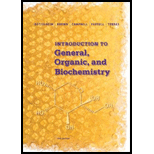# Answer true or false. A hydrocarbon is composed only of the elements carbon and hydrogen. Alkanes are saturated hydrocarbons. The general formula of an alkane is C„H 2 „. 2 , where n is the number of carbons in the alkane, Alkenes and alkynes are unsaturated hydrocarbons.### Introduction to General, Organic a...

11th Edition
Frederick A. Bettelheim + 4 others
Publisher: Cengage Learning
ISBN: 9781285869759### Introduction to General, Organic a...

11th Edition
Frederick A. Bettelheim + 4 others
Publisher: Cengage Learning
ISBN: 9781285869759

#### Solutions

Chapter 11, Problem 11.10P
Textbook Problem

## Answer true or false. A hydrocarbon is composed only of the elements carbon and hydrogen. Alkanes are saturated hydrocarbons.The general formula of an alkane is C„H2„.2, where n is the number of carbons in the alkane,Alkenes and alkynes are unsaturated hydrocarbons.

Expert Solution
Interpretation Introduction

(a)

Interpretation:

Interpret the following statement as true or false.

‘Hydrocarbon composed of carbon and hydrogen‘.

Concept Introduction:

Hydrocarbon contains only carbon and hydrogen. They can be saturated or unsaturated depending upon the type of carbon-carbon bonds. Saturated chain of hydrocarbon contains only single bonds. The carbon in saturated hydrocarbon are joined to each other with single bond and they are sp3 hybridized. Unsaturated hydrocarbons can have double or triple bonds, the carbon atoms in double bond are sp2 hybridized and that in triple bond are sp.

The general formula for alkane is CnH2n+2 which is a saturated hydrocarbon.

The given statement is true.

### Explanation of Solution

The given statement is true because hydrocarbon made of carbon and hydrogen.

The general formula for alkane is CnH2n+2 which is a saturated hydrocarbon. From the general formula, hydrocarbon contains only carbon and hydrogen which are joined to produce long chain of either alkane, alkene or alkyne.

Expert Solution
Interpretation Introduction

(b)

Interpretation:

Interpret the following statement as true or false.

‘Alkanes are saturated hydrocarbon’.

Concept Introduction:

Hydrocarbon contains only carbon and hydrogen. They can be saturated or unsaturated depending upon the type of carbon-carbon bonds. Saturated chain of hydrocarbon contains only single bonds. The carbon in saturated hydrocarbon are joined to each other with single bond and they are sp3 hybridized. Unsaturated hydrocarbons can have double or triple bonds, the carbon atoms in double bond are sp2 hybridized and that in triple bond are sp.

The general formula for alkane is CnH2n+2 which is a saturated hydrocarbon.

The given statement is true.

### Explanation of Solution

Saturated chain of hydrocarbon contains only single bonds. The carbon in saturated hydrocarbon are joined to each other with single bond and they are sp3 hybridized.

A single bonded carbon atom is usually sp3 hybridized and if present in hydrocarbon chain then known as saturated chain of hydrocarbon.

Expert Solution
Interpretation Introduction

(c)

Interpretation:

Interpret the following statement as true or false.

‘General formula of alkane is Cn H2n +2 ’.

Concept Introduction:

Hydrocarbon contains only carbon and hydrogen. They can be saturated or unsaturated depending upon the type of carbon-carbon bonds. Saturated chain of hydrocarbon contains only single bonds. The carbon in saturated hydrocarbon are joined to each other with single bond and they are sp3 hybridized. Unsaturated hydrocarbons can have double or triple bonds, the carbon atoms in double bond are sp2 hybridized and that in triple bond are sp.

The general formula for alkane is CnH2n+2 which is a saturated hydrocarbon.

The given statement is true.

### Explanation of Solution

Methane with molecular formula CH4, ethane with molecular formula C2H6, Propane with molecular formula C3 H8 and butane with molecular formula C4 H10 are the examples of alkane. Their molecular formula indicates the number of hydrogen atoms is 2 more than twice the number of carbon atoms in the structure and thus the derived formula for alkane is Cn H2n +2.

Thus, the general formula for alkane is Cn H2n +2.

Where, n is number of carbon atoms in the principal chain.

Expert Solution
Interpretation Introduction

(d)

Interpretation:

Interpret the following statement as true or false.

‘Alkene and alkyne are unsaturated hydrocarbon’.

Concept Introduction:

Hydrocarbon contains only carbon and hydrogen. They can be saturated or unsaturated depending upon the type of carbon-carbon bonds. Saturated chain of hydrocarbon contains only single bonds. The carbon in saturated hydrocarbon are joined to each other with single bond and they are sp3 hybridized. Unsaturated hydrocarbons can have double or triple bonds, the carbon atoms in double bond are sp2 hybridized and that in triple bond are sp.

The general formula for alkane is CnH2n+2 which is a saturated hydrocarbon.

The given statement is true.

### Explanation of Solution

Unsaturated chain of hydrocarbon contains either double bond or triple bond. The carbon in unsaturated hydrocarbon are joined to each other with double bond known as alkene and they are sp2 hybridized while carbon in unsaturated hydrocarbon are joined to each other with triple bond known as alkyne and they are sp hybridized.

### Want to see more full solutions like this?

Subscribe now to access step-by-step solutions to millions of textbook problems written by subject matter experts!

### Want to see more full solutions like this?

Subscribe now to access step-by-step solutions to millions of textbook problems written by subject matter experts!